# Computing with Curves

by Kirby Urner
First posted: May 2, 2000

The catenary is a curve defined by the hyperbolic cosine (cosh) of (kx), where k is some constant and x is a domain of the function cosh(kx).

In the graph below, x goes from -1 to 1, and k=2. The curve looks a lot like a parabola, but isn't one. The cosh function may be expressed in terms of Euler's number e. We can see this using conventional math notation, and/or Python.

```>>> import math
>>> e = math.e
>>> def g(x): return 0.5*(e**x + e**-x)
>>> g(0)
1.0
>>> g(1)
1.54308063482
```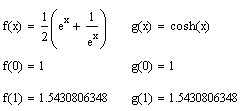Necklaces, overhead telephone lines, anything string-like, hanging from two ends with a dip in the middle owing to the pull of gravity, will tend to assume this catenary shape. On the other hand, when the chain or rope supports the weight of something pulling down evenly along its entire length, like a road bed, in the case of a suspension bridge, then the result is a parabola.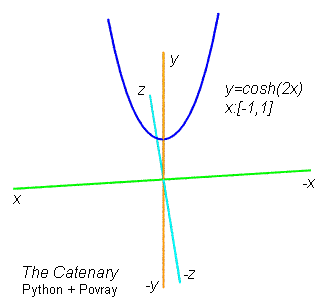The above graphic was developed using Python + Povray, a generic computer language (i.e. Python), and a specialized ray tracing engine with scripting language (i.e. Povray) -- both multi-platform, free downloads. The functions.py source code includes a primitive mkdomain function for returning a list of domain values, from low to high, in constant increments -- (low, high, step) being the 3 parameters passed to this function. Here's mkdomain in action: ```>>> from catenary import * >>> domain = mkdomain(-1,1,0.1) >>> domain [-1.0, -0.9, -0.8, -0.7, -0.6, -0.5, -0.4, -0.3, -0.2, -0.1, 0.0, 0.1, 0.2, 0.3, 0.4, 0.5, 0.6, 0.7, 0.8, 0.9, 1.0] ``` The catenary function gets passed as a rule to mkfunction, along with an optional value for k (which defaults to 1), so that the range values cosh(kx) may be computed for every member of the domain list. ```defcatenary(x,k=1): return math.cosh(k*x) ``` The catenary function returns a list of (domain,range) pairs i.e. a list of tuples. This is what a function amounts to, after all; a function is a set, with each domain value uniquely paired with some corresponding range value, more than it is some rule or algebraic expression. The list of tuples returned by catenary, in turn serves as grist for the mill -- this time for the graphfunc utility. In this function, we take successive (domain,range) points and draw segments connecting them. If the points are close enough together, the locally straight edges nevertheless provide a smoothly curving result. This is how we got the curve shown above. In the case of an overhead caternary railroad, a lower line is suspended from an upper one, and charged with high voltage. This line is then pressed against by the pantograph, that part of a train which makes contact with the overhead wire. In the real world, these might not really be catenary curves -- but these overhead wires are called catenaries, in any case.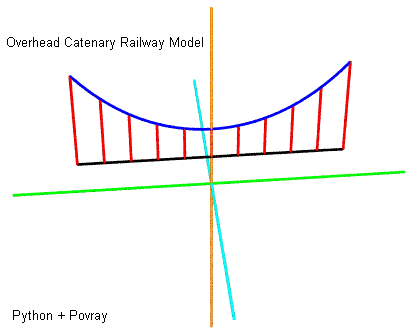Of course it might be useful to figure out how long when of these hanging dips might be. What is the length of a catenary? What is the length of any arc? One solution, given we're doing these Python functions, would be to accumulate the lengths of the little segments we're using to connect the dots: ```defgetlength(myfunc): """ approximates length of curve as sum of segments for list of (x,f(x)) tuples """ length = 0 for i in range(len(myfunc)-1): v1 = Vector((myfunc[i]+(0,))) v2 = Vector((myfunc[i+1]+(0,))) length = length + (v2-v1).length() return length ``` In other words, we're already taking (domain,range) pairs and drawing little segments between successive v1, v2. So let's just write a take the cumulative sum of these lengths and see what we get: ```>>> from functions import * >>> domain = mkdomain(-2,2,0.01) >>> function = mkfunction(domain,catenary,{'k':2.0/3.0}) >>> getlength(function) 4.6476237334964479 >>> domain = mkdomain(-1,1,0.01) >>> function = catenary(domain,catenary,{'k':2.0}) >>> getlength(function) 6.1125198957092381 ``` Notice that it matters how closely together we space our domain points. The closer together they are, the more closely our little segments follow the curve itself. This is a classic example of where the calculus comes in handy, as clearly there's a limit case, where we've gone as far as we can towards bringing our domain points closer together. Using the calculus, we can compute this limiting expression directly, without approximating through a sequence of closer and closer values. The calculus expression for arc length is given below: with low and high values bracketing our domain, we integrate a 2nd root of (1 plus the 2nd power of the derivative function). Given we can take the derivative of cosh(kx), we have an expression for arc length.Notice that L(-2,2,2/3) gives a value rather close to what we got using Python, as does L(-1,1,2). Below we apply the same general approach to obtaining the arc length using a semi-circle instead of a catenary. First, lets specify the function in Python (invoking the already-supplied semicircle rule) and add up all the little segments between successive tuples: ```>>> from functions import * >>> domain = mkdomain(-1,1,0.0001) # passing low, high, interval >>> semicirc = mkfunction(domain,semicircle) >>> getlength(semicirc) 3.1415923595933619 ``` And here's the calculus approach, which nets us the expected result of pi, for the length of a semi-circle with a radius of 1 and a domain of [-1,1]. Note that our discrete math approach in Python came pretty close, given the fine calibration of our increments.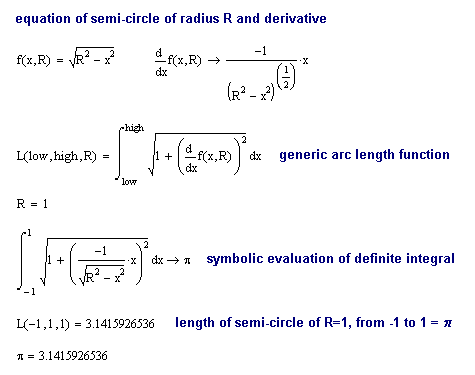We also have a method for computing the tuples of the derivative of some function -- i.e. the (domain, range) pairs of another function. This method wiggles each domain value x by ± h (a parameter), and computes the ratio of the change in f(x) per change in x -- which value approaches the derivative function's value at x, as h becomes arbitrarily small. This derivative function (a set of tuples) may be fed to getlength1, which uses a discrete math version of the above arc length integral, with delta x in place of dx. The value of delta x should be the same as the interval used to compute the original domain. Below, the mkderiv and getlength1 methods are applied to a semi-circle. We use the middle section, from -root(2)/2 to root(2)/2, to avoid troublesome boundary conditions associated with a domain going all the way from -1 to 1. ```>>> from functions import * >>> low = -2**0.5/2.0; high = -low >>> domain = mkdomain(low,high,0.00001) >>> deriv = mkderiv(domain, semicircle, h=0.000001) >>> part = getlength1(deriv,0.00001) # note: same as interval >>> 2 * part # multiply by 2 to get full length of semi-circle 3.1416108619768579 ``` The approximations for length obtained in this way are not as good as using getlength, which in turn falls short of the precision attained by the calculus approach (which depends on symbolic, versus discrete numeric methods to compute the derivative function). Finally, saving the best curve (or at least one of the most popular) for last, we compute the arc length of a parabola over a couple of intervals: [-1,1] and [-2,2]. Note that our indefinite integral for arc length contains the hyperbolic arcsine function (which may be expressed using Ln as shown below). ```>>> import math >>> def asinh(x): return math.log(x+(x**2 + 1)**0.5) >>> asinh(0) 0.0 >>> asinh(1) 0.88137358702 ```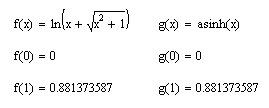The definite integrals evaluate to somewhat simpler expressions however.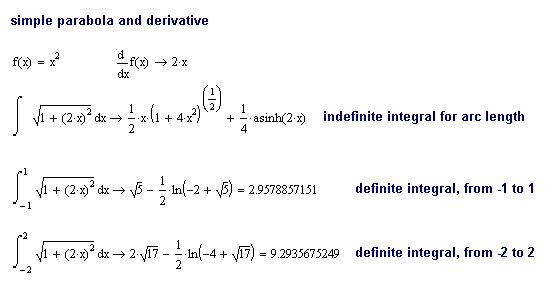Lets see what we get for these lengths using parabola and getlength in our functions module: ```Python 2.0b2 (#6, Sep 26 2000, 14:59:21) [MSC 32 bit (Intel)] on win32 Type "copyright", "credits" or "license" for more information. IDLE 0.6 -- press F1 for help >>> from functions import * >>> domain = mkdomain(-1,1,0.001) >>> function = mkfunction(domain,parabola) >>> getlength(function) 2.9578855660179921 >>> domain = mkdomain(-2,2,0.0002) >>> function = mkfunction(domain,parabola) >>> getlength(function) 9.2935675183982269 ``` The results from computing parabolic arc lengths this way are pretty close, as we should expect. Relevant links: Hyperbolic and inverse hyperbolic functions at S.O.S. Mathematics About the Catenary Catenary and Rolling Polygon (Java animation) Derivation of Arc Length formula St. Louis Arch Suspension Bridge Technical Data Pictures of catenaries (railroad) Re: the hazards of overhead catenaries (railroad) Approximating the length of a parabola (Java applet) Source code is in: python101.zip MathSoft, makers of the MathCad software used in this essay A page of calcs using MathCad (saving as HTML) Postings to Python Edu-Sig: [ 1][ 2][ 3] Computer language index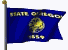Oregon Curriculum Network